## Friday, 8 August 2014

### CHAPTER 8 - Coplanar Vectors

In the preceeding discussion, we talked about the basis of a plane. We can easily extend that discussion to observe that any three non-coplanar vectors can form a basis of three dimensional space:In other words, any vector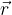$\vec r$ in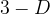$3-D$ space can be expressed as a linear combination of three arbitrary non-coplanar vectors. From this, it also follows that for three non-coplanar vectors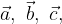$\vec a,\,\,\vec b,\,\,\vec c,$ if their linear combination is zero, i.e, if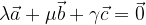$\lambda \vec a + \mu \vec b + \gamma \vec c = \vec 0$ where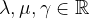${\lambda ,\mu ,\gamma \in\mathbb{R} }$
then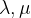$\lambda ,\mu$ and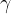$\gamma$ must all be zero. To prove this, assume the contrary. Then, we have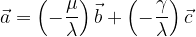$\vec a = \left( { - \dfrac{\mu }{\lambda }} \right)\vec b + \left( { - \dfrac{\gamma }{\lambda }} \right)\vec c$
which means that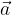$\vec a$ can be written as the linear combination of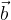$\vec b$ and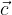$\vec c$. However, this would make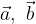$\vec a,\,\,\vec b$ and$\vec c$ coplanar, contradicting our initial supposition. Thus,$\lambda ,\mu$ and$\gamma$ must be zero.
We finally come to what we mean by linearly independent and linearly dependent vectors.
Linearly independent vectors:
A set of non-zero vectors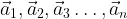${\vec a_1},{\vec a_2},{\vec a_3}\ldots ,{\vec a_n}$ is said to be linearly independent if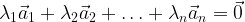${\lambda _1}{\vec a_1} + {\lambda _2}{\vec a_2} + \ldots + {\lambda _n}{\vec a_n} = \vec 0$ implies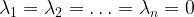${\lambda _1} = {\lambda _2} = \ldots = {\lambda _n} = 0$
Thus, a linear combination of linearly independent vectors cannot be zero unless all the scalars used to form the linear combination are zero.
Linear dependent vectors:
A set of non-zero vectors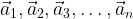${\vec a_1},{\vec a_2},{\vec a_3},\ldots,{\vec a_n}$ is said to be linearly dependent if there exist scalars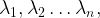${\lambda _1},{\lambda _2}\ldots {\lambda _n},$ not all zero such that,${\lambda _1}{\vec a_1} + {\lambda _2}{\vec a_2} +\ldots + {\lambda _n}{\vec a_n} = \vec 0$
For example, based on our previous discussions, we see that
 (i) Two non-zero, non-collinear vectors are linearly independent. (ii) Two collinear vectors are linearly dependent (iii) Three non-zero, non-coplanar vectors are linearly independent. (iv) Three coplanar vectors are linearly dependent (v) Any four vectors in$3-D$ space are linearly dependent.
You are urged to prove for yourself all these assertions.
 Example: 5
 Let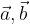$\vec a,\vec b$ and$\vec c$ be non-coplanar vectors. Are the vectors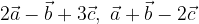$2\vec a - \vec b + 3\vec c,\,\,\vec a + \vec b - 2\vec c$ and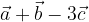$\vec a + \vec b - 3\vec c$ coplanar or non-coplanar?
 Solution: 5
Three vectors are coplanar if there exist scalars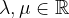$\lambda ,\mu \in \mathbb{R}$ using which one vector can be expressed as the linear combination of the other two.
Let us try to find such scalars: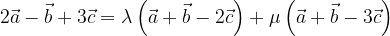$2\vec a - \vec b + 3\vec c = \lambda \left( {\vec a + \vec b - 2\vec c} \right) + \mu \left( {\vec a + \vec b - 3\vec c} \right)$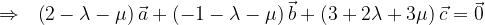$\Rightarrow \,\,\,\, \left( {2 - \lambda - \mu } \right)\vec a + \left( { - 1 - \lambda - \mu } \right)\vec b + \left( {3 + 2\lambda + 3\mu } \right)\vec c = \vec 0$
Since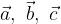$\vec a,\,\,\vec b,\,\,\vec c$ are non-coplanar, we must have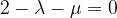$2 - \lambda - \mu = 0$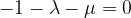$- 1 - \lambda - \mu = 0$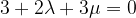$3 + 2\lambda + 3\mu = 0$
This system, as can be easily verified , does not have a solution for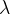$\lambda$ and$\mu$.
Thus, we cannot find scalars for which one vector can be expressed as the linear combination of the other two, implying the three vectors must be non-coplanar.
As an additional exercise, show that for three non-coplanar vectors$\vec a,\,\,\vec b$and$\vec c$, the vectors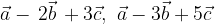$\vec a - \,2\vec b\, + 3\vec c,\,\,\vec a - 3\vec b + 5\vec c$ and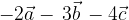$- 2\vec a - \,3\vec b\, - 4\vec c$ are coplanar.